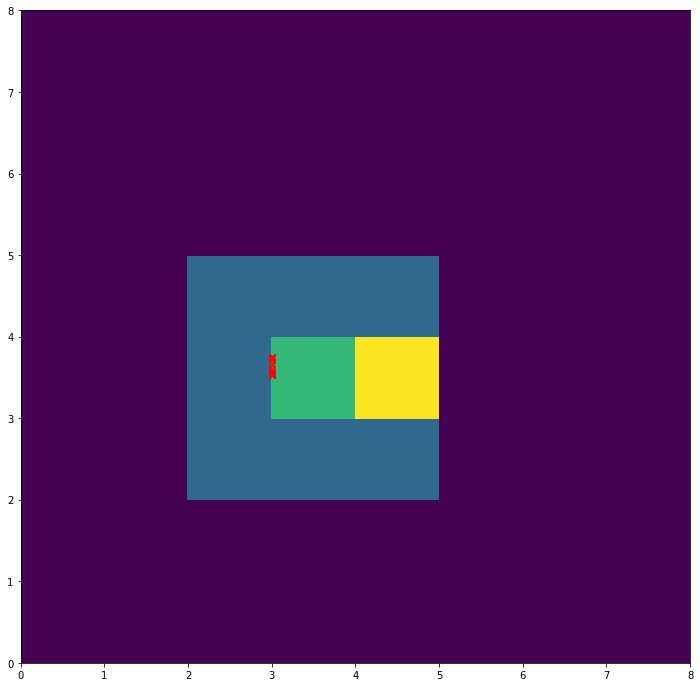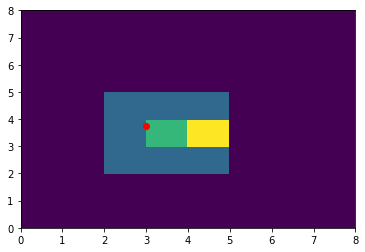In [ ]:
# let's make a test for subpixel localization

In :
import numpy as np
import matplotlib.pyplot as plt
from openpiv.pyprocess import find_first_peak

In :
N = 64

corr = np.zeros((N,N))

corr[2:5,2:5] = 1
corr[3,3] = 2
corr[3,4] = 3
corr[3,5] = 1
corr

Out:
array([[0., 0., 0., ..., 0., 0., 0.],
[0., 0., 0., ..., 0., 0., 0.],
[0., 0., 1., ..., 0., 0., 0.],
...,
[0., 0., 0., ..., 0., 0., 0.],
[0., 0., 0., ..., 0., 0., 0.],
[0., 0., 0., ..., 0., 0., 0.]])
In :
pos,height = find_first_peak(corr)

In :
pos,height

Out:
((3, 4), 3.0)
In :
from openpiv.pyprocess import find_subpixel_peak_position

In :
find_subpixel_peak_position(corr)

Out:
(3.0, 3.769577293545741)

## let's find some corner cases¶

In :
# peak on the border
corr = np.zeros((N,N))

corr[:3,:3] = 1
corr[0,0] = 2
corr[0,2] = 3
corr[0,3] = 1
corr

Out:
array([[2., 1., 3., ..., 0., 0., 0.],
[1., 1., 1., ..., 0., 0., 0.],
[1., 1., 1., ..., 0., 0., 0.],
...,
[0., 0., 0., ..., 0., 0., 0.],
[0., 0., 0., ..., 0., 0., 0.],
[0., 0., 0., ..., 0., 0., 0.]])

## Corner case 1: peak on the border¶

it is disregarded in our function because we cannot define well the subpixel position. Or do we?

In :
find_subpixel_peak_position(corr)

Out:
(nan, nan)
In :
# peak on the border
corr = np.flipud(corr)
corr

Out:
array([[0., 0., 0., ..., 0., 0., 0.],
[0., 0., 0., ..., 0., 0., 0.],
[0., 0., 0., ..., 0., 0., 0.],
...,
[1., 1., 1., ..., 0., 0., 0.],
[1., 1., 1., ..., 0., 0., 0.],
[2., 1., 3., ..., 0., 0., 0.]])
In :
find_subpixel_peak_position(corr)

Out:
(nan, nan)
In :
corr = np.fliplr(corr)
corr[-2,-1]=5
corr

Out:
array([[0., 0., 0., ..., 0., 0., 0.],
[0., 0., 0., ..., 0., 0., 0.],
[0., 0., 0., ..., 0., 0., 0.],
...,
[0., 0., 0., ..., 1., 1., 1.],
[0., 0., 0., ..., 1., 1., 5.],
[0., 0., 0., ..., 3., 1., 2.]])
In :
find_subpixel_peak_position(corr)

Out:
(nan, nan)
In :
## Corner case 2: zero next to the peak - the log(0) fails

In :
corr = np.zeros((N,N))

corr[2:5,2:5] = 1
corr[3,3] = 2
corr[3,4] = 3
# corr[3,5] = 1
corr

Out:
array([[0., 0., 0., ..., 0., 0., 0.],
[0., 0., 0., ..., 0., 0., 0.],
[0., 0., 1., ..., 0., 0., 0.],
...,
[0., 0., 0., ..., 0., 0., 0.],
[0., 0., 0., ..., 0., 0., 0.],
[0., 0., 0., ..., 0., 0., 0.]])
In :
find_subpixel_peak_position(corr)

Out:
(3.0, 3.5230088020336483)
In :
fig, ax = plt.subplots(figsize=(12,12))
ax.pcolor(corr[:8,:8])
for eps in np.logspace(-15,5):
i,j = find_subpixel_peak_position(corr+eps)
# print(i,j)
ax.plot(i,j,'rx')In :
import matplotlib.pyplot as plt

In :
plt.pcolor(corr[:8,:8])
plt.plot(i,j,'ro')

Out:
[<matplotlib.lines.Line2D at 0x7ff38e6d5e20>]In :
for method in ['gaussian','parabolic','centroid']:
i,j = find_subpixel_peak_position(corr,method)
print(i,j)
i,j = find_subpixel_peak_position(corr+eps,method)
print(i,j)

3.0 3.5239508804084627
3.0 3.7499981250225445
3.0 3.75
3.0 3.75
3.0 3.600000095999977
3.0 3.9999933334444426

In [ ]: